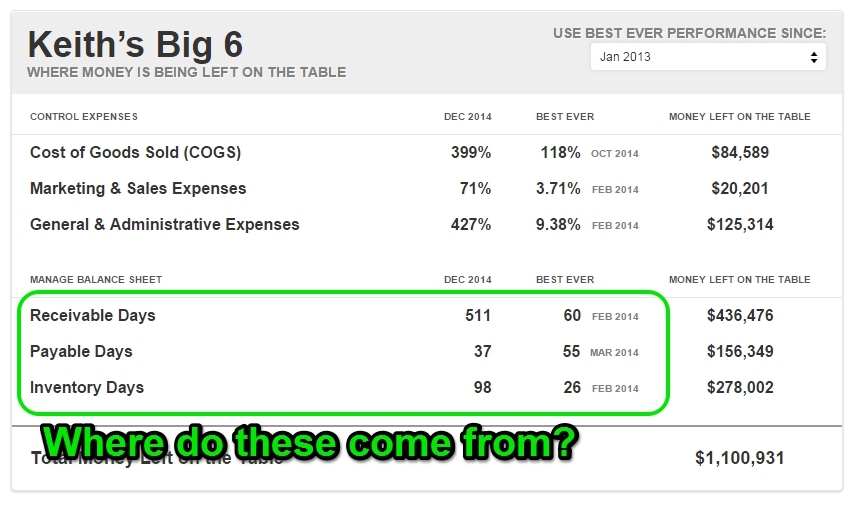# Payable / Receivable / Inventory Days

## How does CFO Scoreboard calculate the "RIP" days shown on the Reports page?

Or in other words:Each of these optics are extremely useful. If you'd like to know more about what they mean, we'd recommend taking a look at our video on Money Left On the Table and Keith's Big 6.

Meanwhile if you want to know how these are calculated, take a look below ...

### Receivable Days

This term is also commonly referred to as Days Sales Outstanding, or DSO.

Receivable Days is calculated on a monthly basis with this formula:

```Receivable Days = (Accounts Receivable) / (Revenue / Days In Month)
```

In plain English it works like this:

1. First we divide total Revenue for the month by the number of days in the month.
2. Then we divide ending Accounts Receivable for the month by the quotient from #1.

Note that in order for this metric to show at all, you must have at least one account classified as Accounts Receivable on the company's  Classify Accounts screen.

### Payable Days

This term is also commonly referred to as  Days Payable Outstanding, or DPO.

Payable Days is calculated on a monthly basis with this formula:

```Payable Days = (Accounts Payable) / ((COGS + Marketing Expenses + G&A Expenses - Payroll) / Days In Month)
```

In plain English it works like this:

1. First we calculate non-payroll expenses by summing together COGS, Marketing Expenses, and G&A, and then subtracting out all accounts that are marked as Payroll expenses.
2. Then we divide the sum from #1 by the  number of days in the month.
3. Finally we divide total ending Accounts Payable for the month by the quotient from #2.

Note that in order for this metric to show at all, you must have at least one account marked respectively as COGS, Marketing & Sales, General & Administrative, Payroll Expense, and Accounts Receivable, on the  Classify Accounts screen.

### Inventory Days

This term is also commonly referred to as  Days Sales of Inventory, or DSI.

Inventory Days is calculated on a monthly basis with this formula:

```Inventory Days = (Inventory) / (COGS / Days In Month)
```

In plain English it works like this:

1. First we divide total COGS for the month by the number of days in the month.
2. Then we divide ending Inventory for the month by the quotient from #1.

Note that in order for this metric to show at all, you must have at least one account classified respectively as COGS and Inventory, on the  Classify Accounts screen.